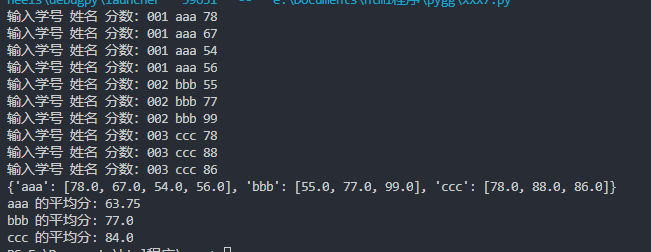2021-06-21 22:29

# Python关于字典的一个问题，求助，谢谢！

• 写回答
• 关注问题
• 收藏
• 邀请回答

#### 3条回答默认 最新

•已采纳

代码如下：{如果对你有帮助，可以给我个采纳吗，谢谢!! 点击我这个回答右上方的【采纳】按钮}。

``````dic = {}
for n in range(10):
a,b,c = input("输入学号 姓名 分数：").strip().split(' ')
c = float(c)
dic.setdefault(b,[]).append(c)
print(dic)
for k,v in dic.items():
avg = sum(v)/len(v)
print(k,"的平均分:",avg)
``````已采纳该答案
1 打赏 评论
•CSDN专家-黄老师 2021-06-21 22:34

学号、姓名和分数都不能作为唯一，而字典的key必须是唯一的，如果不唯一，会覆盖原有的数值，你这个功能有点问题

你可以参考，自己进行修改，可以用时间戳生成key，用做唯一，然后学号、姓名和分数作为字典的value，不过这个判断就非常复杂。

``````a = int(input("请输入学生人数："))
d = {}
for i in range(a):
num = input("成绩为：")
name = input("姓名为：")
d[name] = int(num)
r = sum(d.values()) / len(d.values())
print('平均分：', r)``````

如果对你有帮助，可以点击我这个回答右上方的【采纳】按钮，给我个采纳吗，谢谢

1 打赏 评论
•小P聊技术 2021-06-21 22:42
``````# 1、由用户输入学生学号与姓名，数据用字典存储，最终输出学生信息（按学号由小到大显示）
# 创建字典
students = {}

# 用户输入
student = input("请输入学号：")
ID = input("请输入你的姓名:")

if not(student is None):
students[student] = ID
# 判断是否继续输入
judge = input("是否继续输入(请输入yes继续，输入其他则结束):")

while judge == "yes" or judge == "Yes":
student = input("请输入学号：")
ID = input("请输入你的姓名:")
# 判断学号是否重复,防止更改已输入的信息
while student in students:
if student in students:
print('学号重复请重新输入你的信息')
student = input("请输入学号：")
ID = input("请输入你的姓名:")
else:
break
# 加入字典
students[student] = ID
judge = input("是否继续输入(请输入yes或者no):")
# 排序
list1 = list(students.items())
list1.sort(key=lambda x: x, reverse=False)
print(dict(list1))``````
1 打赏 评论